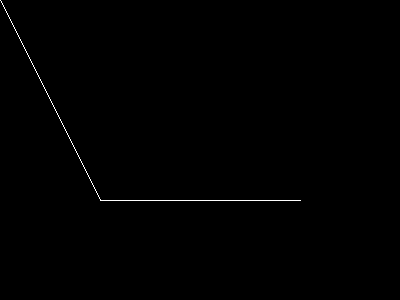# imageopenpolygon

(PHP 7 >= 7.2.0)

imageopenpolygonРисует открытый полигон

### Описание

imageopenpolygon ( resource `\$image` , array `\$points` , int `\$num_points` , int `\$color` ) : bool

imageopenpolygon() рисует открытый полигон на заданном изображении (`image`). В отличие от imagepolygon(), линия между последней и первой точкой не рисуется.

### Список параметров

``` image```

Ресурс изображения, возвращаемый одной из функций создания изображений, например, такой как imagecreatetruecolor().

`points`

Массив, содержащий вершины многоугольника, например:

 points = x0 points = y0 points = x1 points = y1

`num_points`

Общее число точек (вершин), должно быть не меньше 3.

`color`

Идентификатор цвета, созданный функцией imagecolorallocate().

### Возвращаемые значения

Возвращает `TRUE` в случае успешного завершения или `FALSE` в случае возникновения ошибки.

### Примеры

Пример #1 Пример использования imageopenpolygon()

``` <?php// Создать пустое изображение\$image = imagecreatetruecolor(400, 300);// Выделение цвета для полигона\$col_poly = imagecolorallocate(\$image, 255, 255, 255);// Нарисовать полигонimageopenpolygon(\$image, array(        0,   0,        100, 200,        300, 200    ),    3,    \$col_poly);// Вывод изображения в браузерheader('Content-type: image/png');imagepng(\$image);imagedestroy(\$image);?> ```

Результатом выполнения данного примера будет что-то подобное:### Смотрите такжеadd a note

### User Contributed Notes 1 note

-1
marco at oostende dot nl
1 year ago
``` In case you want to use an open polygon but are stuck with a PHP version prior to 7.2, a solution may be to 'backplot' your array to its original start. Say you have an array of pixels (below seperated by commas)<?php\$arr = array();for (\$i = 0; \$i < count(\$pixels); \$i++) {    \$pixel = explode(',', \$pixels[\$i]);    if ((\$pixel > 0) && (\$pixel > 0)) {        \$arr[] = \$pixel;        \$arr[] = \$pixel;    }}imagepolygon(\$im, \$arr, (count(\$arr) / 2), \$otcolor);?>you can replace this by something like<?php\$arr = array();for (\$i = 0; \$i < count(\$pixels); \$i++) {    \$pixel = explode(',', \$pixels[\$i]);    \$arr[] = \$pixel;    \$arr[] = \$pixel;}// imageopenpolygon(\$im, \$arr, (count(\$arr) / 2), \$otcolor) is not possible, so...for (\$i = (count(\$pixels)-1); \$i >= 0; \$i--) {    \$pixel = explode(',', \$pixels[\$i]);    \$arr[] = \$pixel;    \$arr[] = \$pixel;}imagepolygon(\$im, \$arr, (count(\$arr) / 2), \$otcolor);?> ```